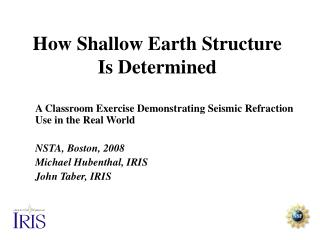# How Shallow Earth Structure Is Determined - PowerPoint PPT PresentationDownload PresentationHow Shallow Earth Structure Is Determined

How Shallow Earth Structure Is DeterminedDownload Presentation## How Shallow Earth Structure Is Determined

- - - - - - - - - - - - - - - - - - - - - - - - - - - E N D - - - - - - - - - - - - - - - - - - - - - - - - - - -
##### Presentation Transcript

1. How Shallow Earth Structure Is Determined A Classroom Exercise Demonstrating Seismic Refraction Use in the Real World NSTA, Boston, 2008 Michael Hubenthal, IRIS John Taber, IRIS

2. Objectives • Explore how refracted seismic waves are used to determine earth structure. • Reinforce the concepts of reflection and refraction of waves through a real world example.

3. Refraction of light

4. Explosion – or energy source C B B1. Layer 1 B2 Layer 2 Prior Knowledge

5. Experiment Design?

6. Distance Direct wave: Slope is 1/v1 Time

7. Distance Direct wave: Slope is 1/v1 Time 1. Measure Direct Wave Slope and you get v1 !!!!

8. Identify: Direct wave Head wave (Refracted wave, upper layer) Refracted wave Reflected wave

9. Direct Wave

10. Direct Wave Reflected Wave

11. Direct Wave Reflected Wave Refracted Waves

12. i1 v1 i2 v2

13. The first head wave is a reflected wave at the critical angle ic ic

14. ic ic The Head wave moves along the surface at the SPEED OF THE LOWER LAYER!!!

15. Distance Direct wave: Slope is 1/v1 Time Head wave: Slope is 1/v2

16. Distance Direct wave: Slope is 1/v1 Time Head wave: Slope is 1/v2 2. Measure Head Wave Slope and you get v2 !!!!

17. What about depth of lower layer?

18. Distance xcross Direct wave: Slope is 1/v1 Time Head wave: Slope is 1/v2 The cross-over distance, xcross , is related to the depth to the boundary!

19. Distance xcross Direct wave: Slope is 1/v1 Time Head wave: Slope is 1/v2 The cross-over distance, xcross , is related to the depth to the boundary!

20. A little geometry and some algebra results in Depth Measure v1 , v2 , and xcross , and you can get the depth to the boundary!!!!

21. Example:

22. Example: Step 1. Find the slope of the Direct wave.

23. Example: Step 2. Find the slope of the Head wave.

24. Example: xcross Step 3. Find the crossover distance

25. Example: xcross Step 3. Find the crossover distance. Step 4. Compute the depth to the boundary.

26. Extensions to simple case

27. EarthScope seismic stations with a good recording of the Magnitude 5.6 earthquake in central California on 10/31/07

28. Depth Upper layer velocity = 6.3 km/s Lower layer velocity = 8.2 km/s Cross over distance = 220 km Depth = 40 km -> Depth to the base of the crust “Moho”